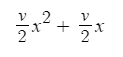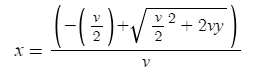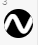# Background

When I was working on a level up system earlier this year, one problem I came across was finding a fast way to calculate how much experience to take and how many levels to give. My original method was to loop over each level and add up the needed experience, but I recently stumbled upon a better method and wanted to share it with anyone looking for a similar solution.

Assuming you are using a linear level system, you should have some kind of "experience points per level" value. For example, I could add 100 experience points on every level up so that each level up requires 100 more experience than the last.

# Steps

### Step 1:

The first thing you should do is model out your level curve. Personally, I recommend desmos. For this method, you should model experience values in terms of how much experience it takes to reach a level from level zero. The line I find that best fits this model is:

``````totalXpNeeded = ((xpPerLevel / 2) * (level ^ 2)) + ((xpPerLevel / 2) * level)
``````

It may look like a mess, so here’s what that looks like in desmos:(Here I’m using v for added experience per level and x for level)

If you would like to use my template for this curve, click here.

So what does this mean, and why is it useful? Well let’s take a look at what we know and don’t know when a player gains experience. We know how much experience they have, and we know how much experience it takes to reach any level from level zero (this is what the function gives you). We don’t know how many times they will level up or how much experience to take yet. What our function allows us to do is solve for them. It’s time to reverse engineer our little formula as a function of experience.

### Step 2:

The goal is to combine your experience and level like some kind of "total experience gained" tracker, where leveling up doesn't take experience (even though it does). You just need a certain amount to reach a given level. How would we do that? Right now we have a function where we can plug in a level and find out the total experience needed to reach it. That's great and all, but we need a function where we can plug in an experience value and see what level you would reach from it. I'm not going to go in depth about the algebra, but when rearranged, the original function can be written like this:
``````level = ((-xpPerLevel / 2) + math.sqrt(((xpPerLevel / 2) ^ 2) + ((xpPerLevel * 2) * totalXp))) / xpPerLevel
``````

I know, another mess. So, I included this on the desmos example as well.(Again, v is added experience per level, and x is level)

Try enabling and disabling the curve with theicon and notice how the two curves overlap. This means that they are the same function, just represented differently.

Alright, the hard part is over. So now what? How do you turn this into something useful?

### Step 3:

This new function allows us to take any experience value and calculate what level a player would be if they started at level zero. Clearly though, players are not going to be level zero, so we need to account for this. Let's substitute in the player's current level into the first function to find what amount of experience they have attained to reach said level, plus how much experience they currently have left over. We'll call this totalXp.
``````local totalXp = ((xpPerLevel / 2) * (level ^ 2)) + ((xpPerLevel / 2) * level) + xp
``````

Now, let’s substitute in the player’s current experience value into our new function to find out what level they would be. Let’s call this new variable predictedLevel

``````local predictedLevel = ((-xpPerLevel / 2) + math.sqrt(((xpPerLevel / 2) ^ 2) + ((xpPerLevel * 2) * totalXp))) / xpPerLevel
``````

Depending on your preference, you may not want decimal levels in your game. You also might want a level cap. We can use math.floor and math.min to achieve both goals:

``````local predictedLevel = math.min(math.floor(((-xpPerLevel / 2) + math.sqrt(((xpPerLevel / 2) ^ 2) + ((xpPerLevel * 2) * totalXp))) / xpPerLevel), levelCap)
``````

This is getting ridiculous. Really hard to read, right? That’s why I recommend desmos. It will save you the headache.

So now we have a predicted level, and the total experience gained since the last level up. The only thing left to do is to actually level up. To check for this, test if the predicted level is greater than the player’s current level.

``````local levelsGained = predictedLevel - level
if levelsGained > 0 then
-- level up
end
``````

In this case, we need to take the right amount of experience and give the player their new level. Luckily for us, this is easy with our two functions. The experience we need to take is equal to the experience needed to reach the predicted level minus the experience needed to reach the current level. Something like this:

``````-- same function from earlier
function GetRequiredXP(level)
return ((xpPerLevel / 2) * (level ^ 2)) + ((xpPerLevel / 2) * level)
end

local xpToCurrentLevel = GetRequiredXP(level.Value)
local totalXp = xpToCurrentLevel + xp.Value
local predictedLevel = math.min(math.floor(((-xpPerLevel / 2) + math.sqrt(((xpPerLevel / 2) ^ 2) + ((xpPerLevel * 2) * totalXp))) / xpPerLevel), levelCap)
local levelsGained = predictedLevel - level.Value
if levelsGained > 0 then
local xpTaken = GetRequiredXP(predictedLevel) - xpToCurrentLevel
xp.Value -= xpTaken
level.Value = predictedLevel
end
``````

And there you have it! My weird and probably overcomplicated way of doing a level up system. If you have any questions or find something wrong with my math, leave a reply for me. Hope this helps!

68 Likes

Good job! This thread is highly empty and underrated. I may even use this myself.

4 Likes

This would be a great way of creating an RPG levelling system, keep up the good work!